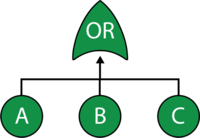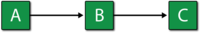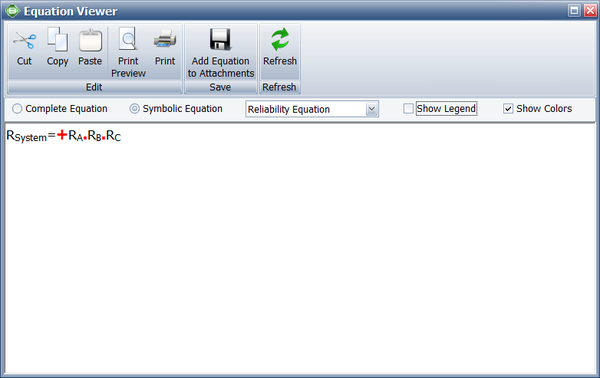# OR Gate Example

This example also appears in the System Analysis Reference book.

### OR Gate

In an OR gate, the output event occurs if at least one of the input events occurs. In system reliability terms, this implies that if any component fails (input) then the system will fail (output). When using RBDs, the equivalent is a series configuration.#### Example

Consider a system with three components, A, B and C. The system fails if A, B or C fails. Draw the fault tree and reliability block diagram for the system. The next two figures show both the FTD and RBD representations.The reliability equation for either configuration is:

${{R}_{System}}={{R}_{A}}\cdot {{R}_{B}}\cdot {{R}_{C}}\,\!$

The figure below shows the analytic equation from BlockSim.